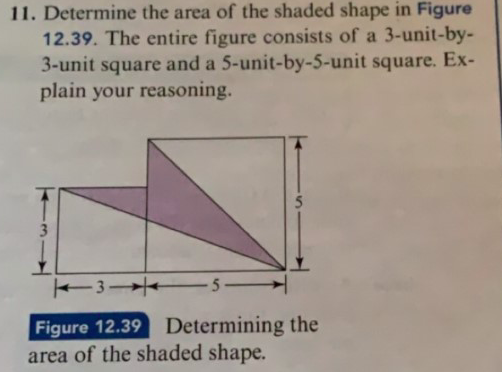# 11. Determine the area of the shaded shape in Figure12.39. The entire figure consists of a 3-unit-by-3-unit square and a 5-unit-by-5-unit square. Ex-plain your reasoning.535_Figure 12.39area of the shaded shape.Determining the

Question
3 viewshelp_outlineImage Transcriptionclose11. Determine the area of the shaded shape in Figure 12.39. The entire figure consists of a 3-unit-by- 3-unit square and a 5-unit-by-5-unit square. Ex- plain your reasoning. 5 3 5_ Figure 12.39 area of the shaded shape. Determining the fullscreen
check_circle

Step 1

Consider the given figure.

Step 2

Since, the combined area of two squares is equal to the sum of the areas of the shaded region and the areas of the unshaded triangles.

So, the unshaded triangle on the left below has height 3 units and base 8 units and the unshaded triangle on the right above has height 5 units and base 5 units.

Step 3

Formula us...

### Want to see the full answer?

See Solution

#### Want to see this answer and more?

Solutions are written by subject experts who are available 24/7. Questions are typically answered within 1 hour.*

See Solution
*Response times may vary by subject and question.
Tagged in

### Mensuration